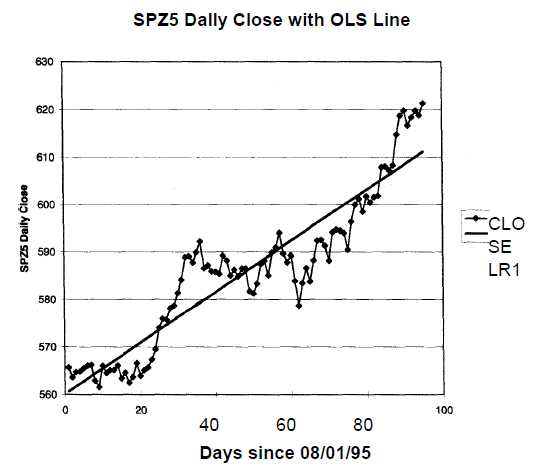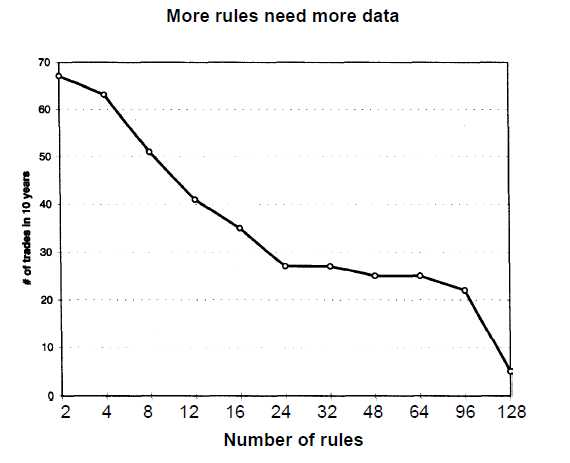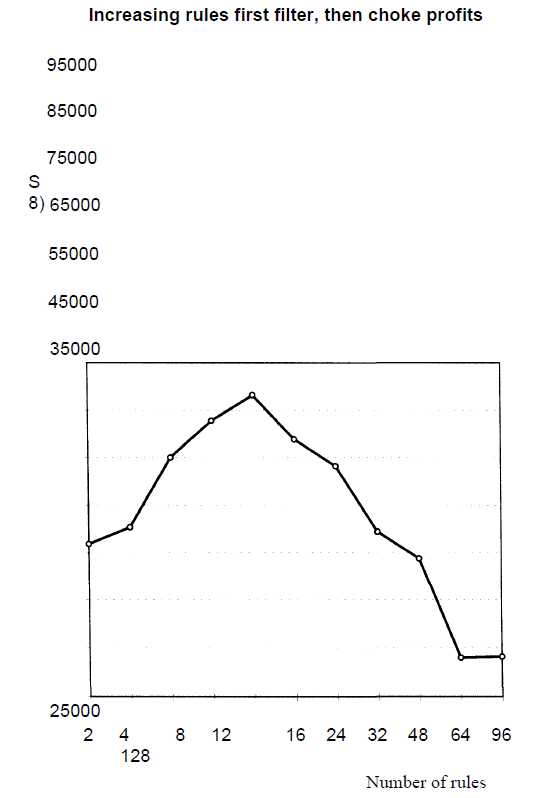# Trading System Rule 2 : A Small Number of Rules

No comment 285 views
5 / 5 ( 1votes )

This article deals with deterministic trading systems using a small number of rules or variables. These trading systems are similar to systems people have developed for tasks such as controlling a chemical process. Their experience suggests that robust, reliable control systems have as few variables as possible.

Trading System Rule 2 : A Small Number of Rules

Consider two well-known trend-following systems. The common dual moving-average system has just two rules. One says to buy the upside crossover, and the other says to sell the downside crossover. Similarly, the popular 20-bar breakout system has at least four rules, two each for entries and exits. You can show with testing software that these systems are profitable over many markets across multiyear time frames.

You can contrast this approach with an expert system-based trading system that may have hundreds of rules. For example, one commercially available system apparently has more than 400 rules. However, it turns out that only one rule is the actual trigger for the trades. The deterministic systems differ from neural-net-based systems that may have an unknown number of rules.
The statistical theory of design of experiments says that even complex processes are controllable using five to seven “main” variables. It is rare for a process to depend on more than ten main variables, and it is quite difficult to reliably control a process that depends on 20 or more variables. It is also rare to find processes that depend on the interactions of four or more variables. Thus, the effect of higher-order interactions is usually insignificant. The goal is to keep the overall number of rules and variables as small as possible.

There are many hazards in designing trading systems with a large number of rules. First, the relative importance of rules decreases as the number of rules increases. Second, the degrees of freedom decrease as the number of rules or variables increases. This means larger amounts of test data are needed to get valid results as the number of rules or variables increases.
A third problem is the danger of curve-fitting the data in the test sample. For example, given a data set, a simple linear regression with just two variables may fit the data adequately. As the number of variables in the regression increases to, say, seven, the line fits the data more closely. Therefore, we can pick up nuances in the data when we curve-fit our trading system, only to pick up patterns that may never repeat in the future. The total degrees of freedom decrease by two for the simple linear regression, but will decrease by seven for the polynomial regression.

These ideas can be illustrated by using regression fits of daily closing data for the December 1995 Standard and Poors 500 (S&P-500) futures contract. The data set covers 95 days from August 1, 1995, through December 13, 1995. Two regression lines are fitted to the same data: Figure 2.1 presents a simple linear regression; Figure 2.2 fits higher-order polynomial terms, going out to the fifth power. As higher-order terms are added, the regression line becomes a curve, and we pick up more nuances in the data.

For simplicity, the daily closes are numbered 1 through 95 and denoted by D. All numbers represented by C (such as Ci) are constants. Est Close is the closing price estimated from the regression.Figure 2.1 SScP-500 closing data with simple linear regression straight line.Figure 2.2 SScP-500 closing data with regression using terms raised to the fifth power.

Est Close = Co + (Ci x D)                                                                                                   (2.1)
Est Close = Co + (Ci x D) + (C^ x D2) + (Cj x D3) + (C4 x D4) + C; x D5)             (2.2)

Table 2.2 illustrates several interesting features about curve-fitting a data set. First, observe that the value of the constant Co is approximately the same for each equation. This implies that the simplest model, the constant Co, captures a substantial amount of information in the data set.

Then, notice that the absolute value of the constants decreases as the order of the term increases. In other words, in absolute value, Co is greater than Ci, which is greater than C2 and on down the line. There-fore, the relative contribution of the higher-order polynomial terms be-comes smaller and smaller. However, as you add the higher-order polynomial terms, the line takes on greater curvature and fits the data more closely, as seen in Figures 2.1 and 2.2.Table 2.2 Comparison of linear regression coefficients

This exercise illustrates many important ideas. First, any model you build for the data should be as simple as possible. In this case, the simple linear regression, with a slope and intercept, captured essentially all the information in the data. Second, adding complexity by adding higher-order terms (read rules) does improve the fit with the data. Thus, we pick up nuances in the data as we build more complex models. The probability that these nuances will repeat exactly is very small. Third, the purpose of our models is to describe how prices have changed over the test period. We used our data to directly calculate the linear regression coefficients. Thus, our model is hostage to the data set. There is no reason why these coefficients should accurately describe any future data. This means that over-fitted trading systems are unlikely to perform as well in the future.

Another example, a variant of the moving-average crossover system, illustrates why it makes sense to limit the number of rules. In the usual case, the dual moving average system has just two rules. For example, for the long entry the 3-day average should cross over the 65-day average and vice versa.

Now, consider a variant that uses more than two averages. For example, buy on the close if both the 3-day and the 4-day moving averages are above the 65-day average. Since there are two “short” averages, this gives us four rules, two each for long and short trades. Using more and more “short” averages rapidly increases the number of rules. For example, if the 3-, 4-, 5-, 6-, and 7-day moving averages should all be above the 65-day average for the long entry, ten rules would apply.

Consider 10 years of Swiss franc continuous contract data, from January 1, 1985, through December 31, 1994, without any initial stop, but allowing \$100 for slippage and commissions. The number of rules is varied from 2 to 128 to explore the effects of increasing the number of rules. As the number of rules increases, the number of trades decreases, as shown in Figure 2.3. This illustrates the fact that as you increase the number of rules, you need more data to perform reliable tests.Figure 2.3 Adding rules reduced the number of trades generated over 10 years of Swiss franc data. Note that the horizontal scale is not linear.

Figure 2.4 shows that the profit initially increased as we added more rules. This means that the extra rules first act as filters and elimi-nate bad trades. As we add even more rules, however, they choke off profits and moreover increase equity curve roughness. Thus, you should be careful to not add dozens of rules.

As stated, this example did not include an initial stop. Hence, as we increase the number of rules, the maximum intraday drawdown should increase because both entries and exits are delayed. You can verify this by using Figure 2.5, page 23.

Calculations for the U.S. bond market from January 1, 1975, through June 30, 1995, illustrate that the general pattern still holds. Figure 2.6, page 24, shows that as the number of rules increases, the profits decrease. The exact patterns will depend on the test data. Data from other markets confirm that increasing rules decreases profits.

Thus, adding rules does not produce endless benefits. Not only do you need more data, but the rising complexity may lead to worsening system performance. A complex system with many rules merely captures nuances within the test data, but these patterns may never repeat. Hence, relatively simple systems are likely to perform better in the future.Figure 2.4 Adding rules increased profits moderately on 10-years of Swiss franc continuous contracts from January 1, 1985, through December 31, 1994. Note that the horizontal scale is not linear.Forex4Live.com is a forex trading system website that started to be published since 2011 and has been used by thousands of traders around the world. The system is very simple and accurate, priced at \$ 167 for a lifetime membership with no monthly fees or hidden fees. Our team created this system by testing thousands of indicators then selecting 23 indicators and combining them into one great trading system.
No Response